# Creating the Equilibrium Manifold¶

For the mass conservation approach of [OMYS17] there are multiple ways that one can form the equilibrium manifold,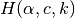. In the approach we have constructed, we have chosen the equilibrium manifold that will result in two characteristics. The first of which is that the decision vector ultimately chosen will consist of only kinetic constants and species’ concentrations. The reason for this is that we would like to remove the need for the user to provide bounds on the so called deficiency parameters,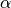. These bounds in practice can be somewhat difficult to find as they are not tied to any physical aspect of the network. The second characteristic we impose is that the manifold will be as close as possible to being linear with respect to the deficiency parameters and those species not in the decision vector. If the manifold is close to being linear in these variables, then solving for them is much simpler, resulting in a shorter solve time for SymPy’s solve function and the avoidance of unsolvable instances of the problem.

We now describe the process taken to find the decision vector and resulting equilibrium manifold. As stated in [OMYS17], the choice of the decision vector is as follows: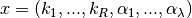for proper and over-dimensioned networks

and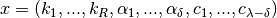for under-dimensioned networks.

Although these decision vectors can be used, it is apparent that if they are chosen then the user will need to provide bounds for the deficiency parameters,. However, as can be inferred by the statement on the bottom of page 7 in the S1 Appendix of [OMYS17], as long as the parametersand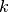are fixed and we can form equation (2.7), then the results of Proposition 1 of page 8 follow. This allows one to choose the decision vector to be as follows for proper and over/under - dimensioned networks: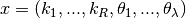,

where the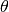values are nonidentical choices of the species’ concentrations,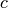.

Now that we have reformed the decision vector to be in terms of just kinetic constants and species’ concentrations, the next step is to choose thevalues such that the equilibrium manifold is as close to being linear as possible. To do this, we first generate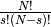choices ofvalues using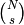, where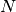is the number of species and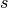is the rank of the stoichiometric matrix. Using each of these sets ofvalues, we then test how many rows of (9) in [OMYS17] are linear in those species’ concentrations that are not inby testing if the second order derivatives of the expression in the row is zero. This is essentially testing if the expression is jointly linear with respect to a given set of species’ concentrations not in.

In practice going through allchoices can be expensive for large networks, to reduce this runtime we exit this routine if all rows of (9) are linear in those species’ concentrations not inand choose this set ofvariables for our decision vector. After choosing the set ofvariables, we then chooseby selecting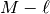independent rows of (9). This process of selectingis reflected in the run of crnt4sbml by the following output produced by crnt4sbml.CRNT.get_mass_conservation_approach()

Creating Equilibrium Manifold ...
Elapsed time for creating Equilibrium Manifold: xx


Once we have selected the equilibrium manifold, we then use the manifold to solve for all the deficiency parameters and species’ concentrations not inusing SymPy’s solve function. This allows us to create expressions for each species’ concentration. This process may take several minutes.